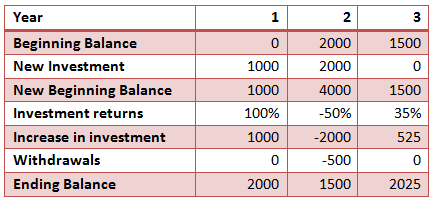# Money-weighted Returns

We learned about arithmetic returns and geometric returns. However, the problem with these measures is that they do not consider the amount of investment made in each period. For example, in the first year, we may have an investment of USD 5,000 while in the second year the investment may only be $2,000. So, the returns when looked at along with how much money was invested will make a huge difference to our actual return on investment. This is called money-weighted return or internal rate of return. Let’s say we had the following investments and returns in the past 3 years: In the first year, we made an investment of$1000, and we had a 100% return in the first year. By the end of the year, our investment has grown to $2,000. Then at the beginning of the second year we invested$2000 more making a total investment of $4000. The returns in the second year were -50%, and our investment value reduced to$2000. Then assume we withdrew $500 from the investment fund, leaving only$1500 invested. In the third year there was no new investment, and our returns were 35%, making our investment grow to $2025. The cash flows are shown in the table below.The money-weighted returns can be calculated using the same formula as that of the Internal rate of Return (IRR).Our cash flows are as follows: CF0 = -$1,000

CF1 = -$2,000 CF2 = +$500